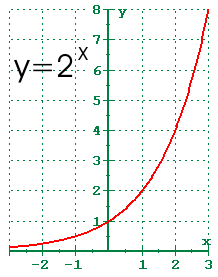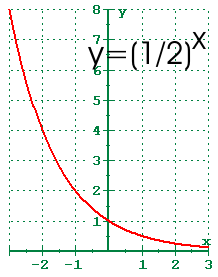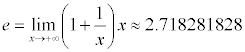# 4.1 - Exponential Functions and Their Graphs

## Exponential Functions

So far, we have been dealing with algebraic functions. Algebraic functions are functions which can be expressed using arithmetic operations and whose values are either rational or a root of a rational number. Now, we will be dealing with transcendental functions. Transcendental functions return values which may not be expressible as rational numbers or roots of rational numbers.

Algebraic equations can be solved most of the time by hand. Transcendental functions can often be solved by hand with a calculator necessary if you want a decimal approximation. However when transcendental and algebraic functions are mixed in an equation, graphical or numerical techniques are sometimes the only way to find the solution.

The simplest exponential function is: f(x) = ax, a>0, a≠1

The reasons for the restrictions are simple. If a≤0, then when you raise it to a rational power, you may not get a real number. Example: If a=-2, then (-2)0.5 = sqrt(-2) which isn't real. If a=1, then no matter what x is, the value of f(x) is 1. That is a pretty boring function, and it is certainly not one-to-one.

Recall that one-to-one functions had several properties that make them desirable. They have inverses that are also functions. They can be applied to both sides of an equation.

## Graphs of Exponential FunctionsThe graph of y=2x is shown to the right. Here are some properties of the exponential function when the base is greater than 1.

• The graph passes through the point (0,1)
• The domain is all real numbers
• The range is y>0.
• The graph is increasing
• The graph is asymptotic to the x-axis as x approaches negative infinity
• The graph increases without bound as x approaches positive infinity
• The graph is continuous
• The graph is smooth

What would the translation be if you replaced every x with -x? It would be a reflection about the y-axis. We also know that when we raise a base to a negative power, the one result is that the reciprocal of the number is taken. So, if we were to graph y=2-x, the graph would be a reflection about the y-axis of y=2x and the function would be equivalent to y=(1/2)x.The graph of y=2-x is shown to the right. Properties of exponential function and its graph when the base is between 0 and 1 are given.

• The graph passes through the point (0,1)
• The domain is all real numbers
• The range is y>0.
• The graph is decreasing
• The graph is asymptotic to the x-axis as x approaches positive infinity
• The graph increases without bound as x approaches negative infinity
• The graph is continuous
• The graph is smooth

Notice the only differences regard whether the function is increasing or decreasing, and the behavior at the left hand and right hand ends.

## Translations of Exponential Graphs

You can apply what you know about translations (from section 1.5) to help you sketch the graph of the exponential functions.

A horizontal translation may affect the increasing / decreasing (if multiplied by a negative), the left hand / right hand behavior of the graph, and the y-intercept, but it won't change the location of the horizontal asymptote.

A vertical translation may affect the increasing / decreasing (if multiplied by a negative), the y-intercept, and the location of the horizontal asymptote. It will not change whether the graph goes without bound or is asymptotic (although it may change where it is asymptotic) to the left or right.

By knowing the features of the basic graphs, you can apply those translations to easily sketch the new function.

You should now add the exponential graph from the front cover of the text to the list of those you know.

## The natural base e

As x increases without bound, the quantity (1+1/x)^x will approach the transcendental number e.The limit notation shown is from calculus. The limit notation is a way of asking what happens to the expression as x approaches the value shown. The limit is the dividing line between calculus and algebra. Calculus is algebra with the concept of limit. People always have this dread of calculus that I can't understand. The calculus itself is easy. The reason people don't do well in calculus is not because of the calculus, but because of they are poor at algebra.

The value for e is approximately 2.718281828. Here is a slightly more accurate, but no more useful, approximation.

2.71828 18284 59045 23536 02874 71352 66249 77572 47093 69995 95749 66967 62772 40766 30353 54759 45716 82178 52516 64274 27466 39193 20030 59921 81741 35966 29043 57290 03342 95260 59563 07381 32328 62794 34907 63233 82988 07531 95251 01901 15738 34187 93070 21540 89149 93488 41675 09244 76146 06680 82264 80016 84774 11853 74234 54424 37107 53907 77449 92069 55170 27618 38606 26133

When the base is e used, the exponential function becomes f(x) = ex. There is a key on your calculator labeled e^x. On the TI-8x calculators, it is on the left side as a [2nd] [Ln]. The exponential function with base e is sometimes abbreviated as exp(). One common place this abbreviation appears is when writing computer programs. I mention this so when I write exp(x), you know what I'm talking about.

## Compounded Interest

The amount in your savings account can be figured with exponential functions. Each period (I'll assume monthly), you get 1/12 of the annual interest rate (r) applied to your account. The new amount in the account is 100% of what you started with plus r%/12 of what you started with that. That means that you now have (100%+r%/12) of what you started with. The next month, you will have the same thing, except it will be based on what you had at the end of the first month.

Confusing, I know. There is an explanation on page 304 of the text, but the resulting formula for compound interest is A = P (1+i)n.

A is the Amount in the account. P is the principal you started with. i is the periodic rate, which is the annual percent (written as a decimal) r, divided by the number of periods per year, m. n is the number of compounding periods, which is equal to the number of periods per year, m, times the time in years, t. The formula I have shown above differs slightly from the formula in the book, but agrees with the formula that you'll use if you go on to Finite Mathematics (Math 160). In Finite Mathematics, there is an entire chapter on finance and the formulas involved.

## Continuous Compounding and Growth / Decay

In the old days, there used to be continuous compounding of interest. You don't find it any more because it is gives the highest return on the investment, and banks are in business to make money, just like any other for profit institution.

The model for continuous compounding is A = P ert.

A is the Amount, P is the Principal, r is the annual percentage rate (written as a decimal), and t is the time in years. e is the base for natural logarithms.

However, the continuous model does make sense for population growth and radioactive decay. The radioactivity of an isotope doesn't change once a month at the end of the month, it is continually changing.

The exponential model is y = A ekt,

where y is the amount present at time t. A is the initial amount present, and k is the rate of growth (if positive) or the rate of decay (if negative).